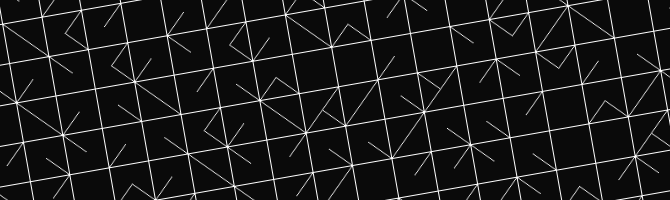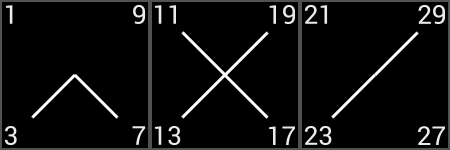# zsmb's prog site

## Prime Table Generator

##### 2015.01.26.### Introduction

The origin story of this project goes back approximately 10 years, when on a math competition, I spent an evening copying a weird-looking sheet of paper, lent to me by a professor whose name unfortunately I don't remember. Any searches I made for the origins of this table didn't turn up anything either. With that said, here's a  picture of my original on paper, before I explain what this is and how it works.

### Description

Let's get into the explanation part. This table contains all primes below 4000 (with the exception of 2 and 5). The upper left square represents the numbers 0-9, the one on its right the numbers 10-19, and so on. In the original 20 by 20 square version, this means that the second row starts at 200, as marked on the paper.

Now, for the prime part of things. With the exception of the aforementioned missing primes, all others end on the digits 1, 3, 7 or 9. In each square, a corner represents one of these digits, the upper left corner being 1, and then going counter-clockwise around the center of the square. If the number belonging to that corner of the square is a prime, there is a line connecting the corner with the center of the square.

As an example, here are the first three squares of the first row with numbers, showing us that 3, 7, 11, 13, 17, 19, 23 and 29 are the first few primes (again, with the exception of 2 and 5, for the obvious reasons).While this is by no means an efficient way to look up whether a number is prime or not (although it's not that slow), it's an interesting riddle to solve if the rows and columns aren't numbered.